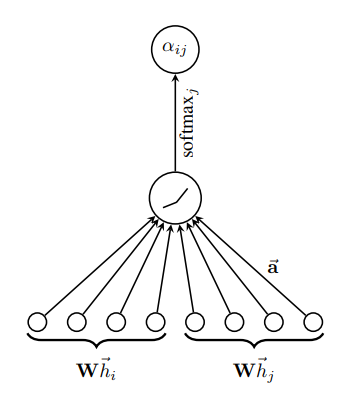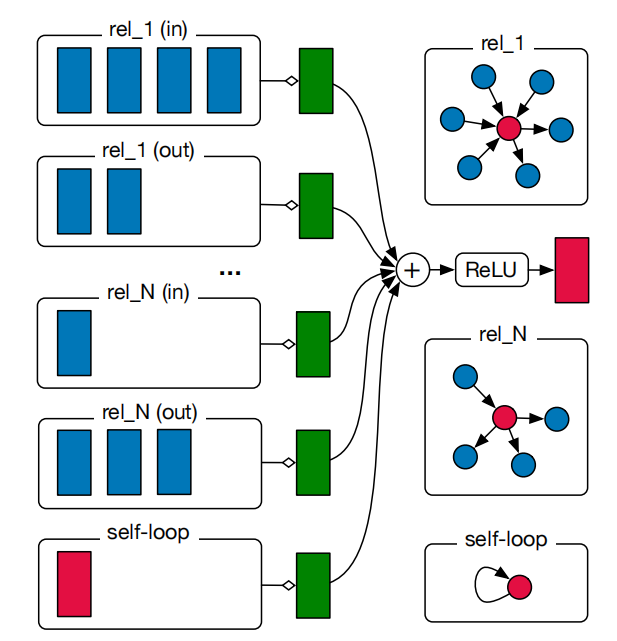# 图神经网络学习笔记：从GCN到GAT再到Relation-aware GNN

## 图神经网络的一般框架

$m_i^k=\sum_{i \in N(j)} \boldsymbol{M}_k(\boldsymbol{H}^{k-1}_i, \boldsymbol{H}^{k-1}_j, e_{ij}) \\ \boldsymbol{H}^k_i=\boldsymbol{U}_k(\boldsymbol{H}_i^{k-1},m_i^k)$

$\boldsymbol{M}_k(\cdot,\cdot,\cdot)$表示消息函数，定义了第 $k$ 层中节点 $i$ 和节点 $j$ 的消息，其取决于第 $k-1$ 层的节点特征（$\boldsymbol{H}^{k-1}_i, \boldsymbol{H}^{k-1}_j$）以及这两个节点间的关系强度 $e_{ij}$。（$N(j)$表示节点 $j$ 的邻居节点）

$\boldsymbol{U}_k(\cdot,\cdot)$是第 $k$ 层的节点更新函数，其结合节点本身的特征和邻居节点的特征进行消息聚合。

## 图卷积网络（GCN）

GCN核心公式如下：

$\boldsymbol{H}^k_i=\sigma(\sum_{j\in N(i)} \frac{\boldsymbol{A}_{ij}}{\sqrt{\boldsymbol{\tilde{D}}_{ii}\boldsymbol{\tilde{D}}_{jj}}}\boldsymbol{H}^{k-1}_j \boldsymbol{W}^k + \frac{1}{\boldsymbol{\tilde{D}}_{i}}\boldsymbol{H}^{k-1}_i \boldsymbol{W}^k )$

## 图注意力网络（GAT）

$e_{ij}=a(\boldsymbol{W}\boldsymbol{H}^{k-1}_{i}, \boldsymbol{W}\boldsymbol{H}^{k-1}_{j})$## Relational Graph Convolutional Network（R-GCN）

R-GCN就是在GCN的基础上进行改进，添加了对边种类进行建模的模型。将GCN和R-GCN的核心公式对比如下：

$GCN: \boldsymbol{H}^k_i=\sigma(\sum_{j\in N(i)} \frac{\boldsymbol{A}_{ij}}{\sqrt{\boldsymbol{\tilde{D}}_{ii}\boldsymbol{\tilde{D}}_{jj}}}\boldsymbol{H}^{k-1}_j \boldsymbol{W}^k + \frac{1}{\boldsymbol{\tilde{D}}_{i}}\boldsymbol{H}^{k-1}_i \boldsymbol{W}^k )$

$RGCN:\boldsymbol{H}^k_i=\sigma(\sum_{r\in R} \sum_{j\in N(i)} \frac{1}{c_{i,r}} \boldsymbol{W}^{k}_r \boldsymbol{H}^{k-1}_j + \boldsymbol{W}^{k}_0 \boldsymbol{H}^{k-1}_i )$Intro to Relational - Graph Convolutional Networks - YouTube